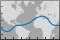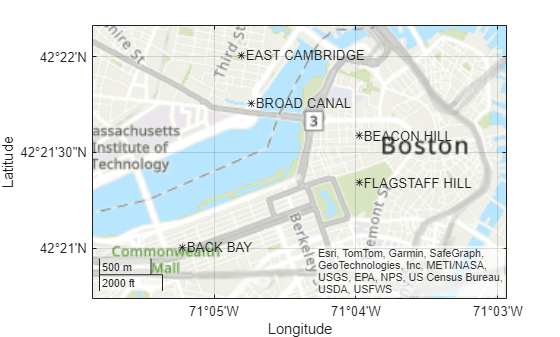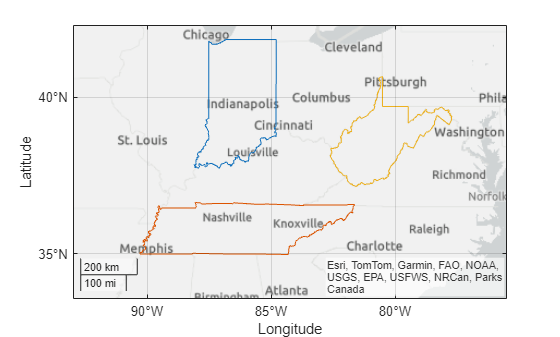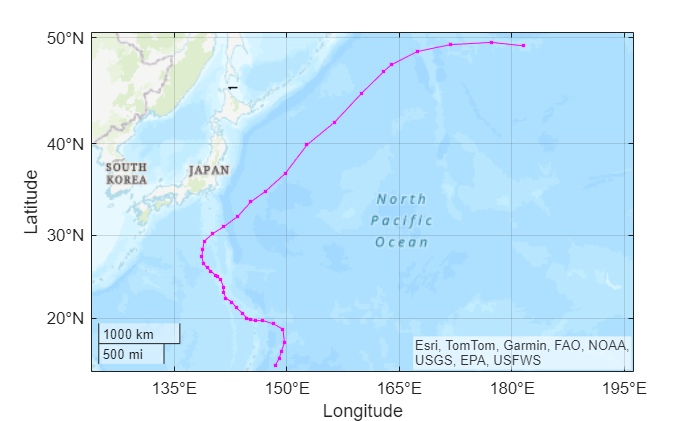# geoplot

Plot line in geographic coordinates

•## Syntax

``geoplot(lat,lon)``
``geoplot(lat,lon,LineSpec)``
``geoplot(lat1,lon1,...,latN,lonN)``
``geoplot(lat1,lon1,LineSpec1,...,latN,lonN,LineSpecN)``
``geoplot(tbl,latvar,lonvar)``
``geoplot(gx,___)``
``geoplot(___,Name,Value)``
``h = geoplot(___)``

## Description

### Vector Data

example

````geoplot(lat,lon)` plots a line in a geographic axes with vertices at the latitude-longitude locations specified (in degrees) by the vectors `lat` and `lon`. The `lat` and `lon` inputs must be the same size. By default, `geoplot` draws a solid line, with no markers, using colors specified by the `ColorOrder` property of the geographic axes object.```

example

````geoplot(lat,lon,LineSpec)` sets the style, marker symbol, and color for the line.```
````geoplot(lat1,lon1,...,latN,lonN)` combines the plots specified by several sets of latitude-longitude locations.```

example

````geoplot(lat1,lon1,LineSpec1,...,latN,lonN,LineSpecN)` combines the plots specified by several sets of latitude-longitude locations, with a separate `LineSpec` for each line.```

### Table Data

example

````geoplot(tbl,latvar,lonvar)` plots the variables `latvar` and `lonvar` from the table `tbl`. To plot one data set, specify one variable for `latvar` and one variable for `lonvar`. To plot multiple data sets, specify multiple variables for `latvar`, `lonvar`, or both. If both arguments specify multiple variables, they must specify the same number of variables. (Since R2022b) ```

````geoplot(gx,___)` plots in the geographic axes specified by `gx` instead of the current axes. ```

example

````geoplot(___,Name,Value)` specifies properties of the chart line using one or more name-value arguments.```
````h = geoplot(___)` returns a column vector of Chart Line objects. Each object corresponds to a plotted line. Use `h` to modify the properties of the Chart line objects. NoteMapping Toolbox™ extends the functionality of the `geoplot` (MATLAB®) function. It adds support for displaying points, lines, and polygons with coordinates in any supported geographic or projected coordinate reference system (CRS). For the `geoplot` (Mapping Toolbox) page, see `geoplot` (Mapping Toolbox). ```

## Examples

collapse all

Plot a straight line between two points on a map. Specify the endpoints of the line using the coordinates of Seattle and Anchorage. Specify latitude and longitude in degrees.

```latSeattle = 47.62; lonSeattle = -122.33; latAnchorage = 61.20; lonAnchorage = -149.9;```

Plot the data on a map. Customize the appearance of the line using the line specification `'g-*'`. Adjust the latitude and longitude limits of the map using `geolimits`.

```geoplot([latSeattle latAnchorage],[lonSeattle lonAnchorage],'g-*') geolimits([45 62],[-149 -123])```Plot a straight line between two points on a map. Specify the endpoints of the line using the coordinates of Seattle and Anchorage. Specify latitude and longitude in degrees.

```latSeattle = 47.62; lonSeattle = -122.33; latAnchorage = 61.20; lonAnchorage = -149.9;```

Plot the data using `geoplot`. Customize the appearance of the line using the line specification `'g-*'`. Adjust the latitude and longitude limits of the map using `geolimits`.

```geoplot([latSeattle latAnchorage],[lonSeattle lonAnchorage],'g-*') geolimits([45 62],[-149 -123])```Identify Anchorage using the `text` function.

`text(latAnchorage,lonAnchorage,'Anchorage');`Identify Seattle using the `text` function. Adjust the alignment of the text label by specifying properties of the `Text` object.

```text(latSeattle,lonSeattle,'Seattle',... 'HorizontalAlignment','right',... 'VerticalAlignment','bottom');```Specify the latitude and longitude coordinates of Seattle, Anchorage, and Point Barrow.

```latSeattle = 47.62; lonSeattle = -122.33; latAnchorage = 61.20; lonAnchorage = -149.9; latPtBarrow = 71.38; lonPtBarrow = -156.47;```

Plot straight lines from Seattle to each of the other two cities. Draw a solid yellow line from Seattle to Anchorage and a dotted blue line between Seattle and Point Barrow. Adjust the latitude and longitude limits of the map using `geolimits`.

```geoplot([latSeattle latAnchorage],[lonSeattle lonAnchorage],'y-',... [latSeattle latPtBarrow],[lonSeattle lonPtBarrow],'b:') geolimits([44 73],[-149 -123])```Label each city on the map using the `text` function.

```text(latAnchorage,lonAnchorage,'Anchorage'); text(latPtBarrow,lonPtBarrow,'Point Barrow'); text(latSeattle,lonSeattle,'Seattle',... 'VerticalAlignment','bottom');```Since R2022b

A convenient way to plot data from a table is to pass the table to the `geoplot` function and specify the variables to plot.

Load a MAT file containing cyclone data into the workspace. Create a table using a subset of the data. The table includes latitude and longitude coordinates in the table variables `Latitude` and `Longitude`, respectively.

```load cycloneTracks tbl = cycloneTracks(cycloneTracks.ID == 1320,:);```

Plot the latitude and longitude coordinates over a topographic basemap. Return the `Line` object as `h`.

```h = geoplot(tbl,"Latitude","Longitude"); geobasemap topographic```

Change the marker style and color of the plot by setting the `Marker` and `Color` properties.

```h.Marker = "."; h.Color = "m";```Plot a straight line between two points on a map. Specify the endpoints of the line using the coordinates of Seattle and Anchorage. Specify latitude and longitude in degrees.

```latSeattle = 47.62; lonSeattle = -122.33; latAnchorage = 61.20; lonAnchorage = -149.9;```

Plot the data using `geoplot`. Adjust the latitude and longitude limits of the map using `geolimits`. Customize the appearance of the line by specifying the `LineWidth` and `Color` properties.

```geoplot([latSeattle latAnchorage],[lonSeattle lonAnchorage],... 'LineWidth',2,'Color',[.6 0 0]) geolimits([45 62],[-149 -123])```Plot a straight line between Seattle and Anchorage on a map. Specify the latitude and longitude coordinates in degrees.

```latSeattle = 47.62; lonSeattle = -122.33; latAnchorage = 61.20; lonAnchorage = -149.9; geoplot([latSeattle latAnchorage],[lonSeattle lonAnchorage])```

Change the latitude-longitude limits and the basemap.

```geolimits([45 62],[-149 -123]) geobasemap colorterrain```## Input Arguments

collapse all

Latitude coordinates in degrees, specified as a real, numeric, finite vector. The vector can contain embedded `NaN`s. `lat` must be the same size as `lon`.

Example: `[43.0327 38.8921 44.0435]`

Data Types: `single` | `double`

Longitude coordinates in degrees, specified as a real, numeric, finite vector. The vector can contain embedded `NaN`s. `lon` must be the same size as `lat`.

Example: `[-107.5556 -77.0269 -72.5565]`

Data Types: `single` | `double`

Line style, marker, and color, specified as a string or character vector containing symbols. The symbols can appear in any order. You do not need to specify all three characteristics (line style, marker, and color). For example, if you omit the line style and specify the marker, then the plot shows only the marker and no line.

Example: `"--or"` is a red dashed line with circle markers

Line StyleDescriptionResulting Line
`"-"`Solid line`"--"`Dashed line`":"`Dotted line`"-."`Dash-dotted lineMarkerDescriptionResulting Marker
`"o"`Circle`"+"`Plus sign`"*"`Asterisk`"."`Point`"x"`Cross`"_"`Horizontal line`"|"`Vertical line`"square"`Square`"diamond"`Diamond`"^"`Upward-pointing triangle`"v"`Downward-pointing triangle`">"`Right-pointing triangle`"<"`Left-pointing triangle`"pentagram"`Pentagram`"hexagram"`HexagramColor NameShort NameRGB TripletAppearance
`"red"``"r"``[1 0 0]``"green"``"g"``[0 1 0]``"blue"``"b"``[0 0 1]``"cyan"` `"c"``[0 1 1]``"magenta"``"m"``[1 0 1]``"yellow"``"y"``[1 1 0]``"black"``"k"``[0 0 0]``"white"``"w"``[1 1 1]`Source table containing the data to plot, specified as a table or a timetable.

Table variables containing the latitude coordinates, specified using one of the indexing schemes from the table.

Indexing SchemeExamples

Variable names:

• `"A"` or `'A'` — A variable called `A`

• `["A","B"]` or `{'A','B'}` — Two variables called `A` and `B`

• `"Var"+digitsPattern(1)` — Variables named `"Var"` followed by a single digit

Variable index:

• An index number that refers to the location of a variable in the table.

• A vector of numbers.

• A logical vector. Typically, this vector is the same length as the number of variables, but you can omit trailing `0` or `false` values.

• `3` — The third variable from the table

• `[2 3]` — The second and third variables from the table

• `[false false true]` — The third variable

Variable type:

• `vartype("categorical")` — All the variables containing categorical values

Regardless of variable names, the axis label on the plot is always `Latitude`.

The variables must contain numeric data of type `single` or `double`. The data must be in the range (–90, 90).

If `latvar` and `lonvar` both specify multiple variables, the number of variables must be the same.

Example: `geoplot(tbl,["lat1","lat2"],"lon")` specifies the table variables named `lat1` and `lat2` for the latitude coordinates.

Example: `geoplot(tbl,2,"lon")` specifies the second variable for the latitude coordinates.

Example: `geoplot(tbl,vartype("numeric"),"lon")` specifies all numeric variables for the latitude coordinates.

Table variables containing the longitude coordinates, specified using one of the indexing schemes from the table.

Indexing SchemeExamples

Variable names:

• `"A"` or `'A'` — A variable called `A`

• `["A","B"]` or `{'A','B'}` — Two variables called `A` and `B`

• `"Var"+digitsPattern(1)` — Variables named `"Var"` followed by a single digit

Variable index:

• An index number that refers to the location of a variable in the table.

• A vector of numbers.

• A logical vector. Typically, this vector is the same length as the number of variables, but you can omit trailing `0` or `false` values.

• `3` — The third variable from the table

• `[2 3]` — The second and third variables from the table

• `[false false true]` — The third variable

Variable type:

• `vartype("categorical")` — All the variables containing categorical values

Regardless of variable names, the axis label on the plot is always `Longitude`.

The variables you specify must contain numeric data of type `single` or `double`.

If `latvar` and `lonvar` both specify multiple variables, the number of variables must be the same.

Example: `geoplot(tbl,"lat",["lon1","lon2"])` specifies the table variables named `lon1` and `lon2` for the longitude coordinates.

Example: `geoplot(tbl,"lat",2)` specifies the second variable for the longitude coordinates.

Example: `geoplot(tbl,"lat",vartype("numeric"))` specifies all numeric variables for the longitude coordinates.

Target geographic axes, specified as a `GeographicAxes` object.1 You can modify the appearance and behavior of a `GeographicAxes` object by setting its properties. For a list of properties, see GeographicAxes Properties.

### Name-Value Arguments

Specify optional pairs of arguments as `Name1=Value1,...,NameN=ValueN`, where `Name` is the argument name and `Value` is the corresponding value. Name-value arguments must appear after other arguments, but the order of the pairs does not matter.

Before R2021a, use commas to separate each name and value, and enclose `Name` in quotes.

Example: `h = geoplot(lat,lon,'Color','g')`

Note

Property settings apply to all the lines in the plot. To set the properties of an individual line, retrieve the handle to the line in the `geoplot` return value `h` and use dot notation.

The name-value pairs listed here are only a subset. For a full list, see Line Properties.

Line color, specified as an RGB triplet, a hexadecimal color code, a color name, or a short name.

For a custom color, specify an RGB triplet or a hexadecimal color code.

• An RGB triplet is a three-element row vector whose elements specify the intensities of the red, green, and blue components of the color. The intensities must be in the range `[0,1]`, for example, ```[0.4 0.6 0.7]```.

• A hexadecimal color code is a character vector or a string scalar that starts with a hash symbol (`#`) followed by three or six hexadecimal digits, which can range from `0` to `F`. The values are not case sensitive. Therefore, the color codes `"#FF8800"`, `"#ff8800"`, `"#F80"`, and `"#f80"` are equivalent.

Alternatively, you can specify some common colors by name. This table lists the named color options, the equivalent RGB triplets, and hexadecimal color codes.

Color NameShort NameRGB TripletHexadecimal Color CodeAppearance
`"red"``"r"``[1 0 0]``"#FF0000"``"green"``"g"``[0 1 0]``"#00FF00"``"blue"``"b"``[0 0 1]``"#0000FF"``"cyan"` `"c"``[0 1 1]``"#00FFFF"``"magenta"``"m"``[1 0 1]``"#FF00FF"``"yellow"``"y"``[1 1 0]``"#FFFF00"``"black"``"k"``[0 0 0]``"#000000"``"white"``"w"``[1 1 1]``"#FFFFFF"``"none"`Not applicableNot applicableNot applicableNo color

Here are the RGB triplets and hexadecimal color codes for the default colors MATLAB uses in many types of plots.

RGB TripletHexadecimal Color CodeAppearance
`[0 0.4470 0.7410]``"#0072BD"``[0.8500 0.3250 0.0980]``"#D95319"``[0.9290 0.6940 0.1250]``"#EDB120"``[0.4940 0.1840 0.5560]``"#7E2F8E"``[0.4660 0.6740 0.1880]``"#77AC30"``[0.3010 0.7450 0.9330]``"#4DBEEE"``[0.6350 0.0780 0.1840]``"#A2142F"`Example: `'blue'`

Example: `[0 0 1]`

Example: `'#0000FF'`

Line style, specified as one of the options listed in this table.

Line StyleDescriptionResulting Line
`"-"`Solid line`"--"`Dashed line`":"`Dotted line`"-."`Dash-dotted line`"none"`No lineNo line

Line width, specified as a positive value in points, where 1 point = 1/72 of an inch. If the line has markers, then the line width also affects the marker edges.

The line width cannot be thinner than the width of a pixel. If you set the line width to a value that is less than the width of a pixel on your system, the line displays as one pixel wide.

Marker symbol, specified as one of the markers in this table. By default, a chart line does not have markers. Add markers at each data point along the line by specifying a marker symbol.

MarkerDescriptionResulting Marker
`"o"`Circle`"+"`Plus sign`"*"`Asterisk`"."`Point`"x"`Cross`"_"`Horizontal line`"|"`Vertical line`"square"`Square`"diamond"`Diamond`"^"`Upward-pointing triangle`"v"`Downward-pointing triangle`">"`Right-pointing triangle`"<"`Left-pointing triangle`"pentagram"`Pentagram`"hexagram"`Hexagram`"none"`No markersNot applicable

Marker fill color, specified as `"auto"`, an RGB triplet, a hexadecimal color code, a color name, or a short name. The `"auto"` option uses the same color as the `Color` property of the parent axes. If you specify `"auto"` and the axes plot box is invisible, the marker fill color is the color of the figure.

For a custom color, specify an RGB triplet or a hexadecimal color code.

• An RGB triplet is a three-element row vector whose elements specify the intensities of the red, green, and blue components of the color. The intensities must be in the range `[0,1]`, for example, ```[0.4 0.6 0.7]```.

• A hexadecimal color code is a character vector or a string scalar that starts with a hash symbol (`#`) followed by three or six hexadecimal digits, which can range from `0` to `F`. The values are not case sensitive. Therefore, the color codes `"#FF8800"`, `"#ff8800"`, `"#F80"`, and `"#f80"` are equivalent.

Alternatively, you can specify some common colors by name. This table lists the named color options, the equivalent RGB triplets, and hexadecimal color codes.

Color NameShort NameRGB TripletHexadecimal Color CodeAppearance
`"red"``"r"``[1 0 0]``"#FF0000"``"green"``"g"``[0 1 0]``"#00FF00"``"blue"``"b"``[0 0 1]``"#0000FF"``"cyan"` `"c"``[0 1 1]``"#00FFFF"``"magenta"``"m"``[1 0 1]``"#FF00FF"``"yellow"``"y"``[1 1 0]``"#FFFF00"``"black"``"k"``[0 0 0]``"#000000"``"white"``"w"``[1 1 1]``"#FFFFFF"``"none"`Not applicableNot applicableNot applicableNo color

Here are the RGB triplets and hexadecimal color codes for the default colors MATLAB uses in many types of plots.

RGB TripletHexadecimal Color CodeAppearance
`[0 0.4470 0.7410]``"#0072BD"``[0.8500 0.3250 0.0980]``"#D95319"``[0.9290 0.6940 0.1250]``"#EDB120"``[0.4940 0.1840 0.5560]``"#7E2F8E"``[0.4660 0.6740 0.1880]``"#77AC30"``[0.3010 0.7450 0.9330]``"#4DBEEE"``[0.6350 0.0780 0.1840]``"#A2142F"`Marker size, specified as a positive value in points, where 1 point = 1/72 of an inch.

## Output Arguments

collapse all

Geographic plot, returned as a column vector of `Line` objects. Each object corresponds to a plotted line. Use `h` to modify the properties of the objects after they are created.

## Tips

• Mapping Toolbox extends the functionality of the `geoplot` (MATLAB) function. It adds support for displaying points, lines, and polygons with coordinates in any supported geographic or projected coordinate reference system (CRS). For the `geoplot` (Mapping Toolbox) page, see `geoplot` (Mapping Toolbox).

• Plot 3-D geographic data using the Mapping Toolbox functions `geoglobe` (Mapping Toolbox) and `geoplot3` (Mapping Toolbox).

• When you plot on geographic axes, the `geoplot` function assumes that coordinates are referenced to the WGS84 coordinate reference system. If you plot using coordinates that are referenced to a different coordinate reference system, then the coordinates may appear misaligned.

• You cannot plot data that requires Cartesian axes in a geographic axes.

• To plot additional data in the geographic axes, use the ```hold on``` command.

## Version History

Introduced in R2018b

expand all